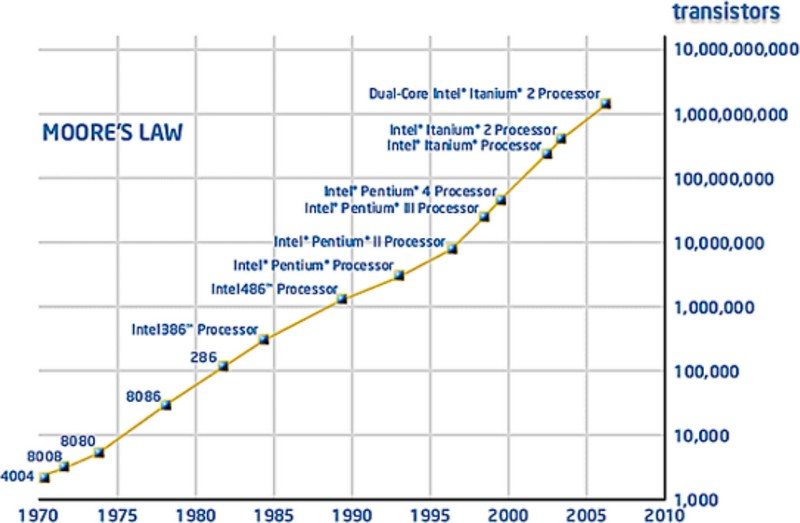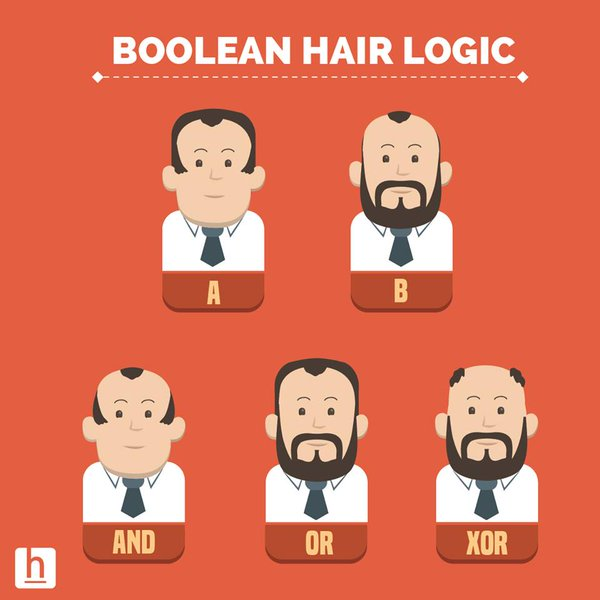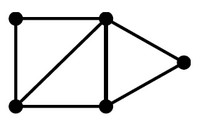# Language - Operator

An operator is a symbol (token) that compose an expression.

It operates on values on its side (left and/or right).

They represents a function but where the argument are on both side of it.

$$ie \\ \text{ }\\ c = a + b \\ \text{ }\\ \text{is equivalent to} \\ \text{ }\\ c = f(a,b)$$

This function are mathematical function (ie they return the same data type than their input)

Operators can also be seen as actions on variables and values.

## Example

### Simple Form

a + b


### Assignment

var += a
// equivalent of
var = var + a


See Assignment

Discover More(Function | Operator | Map | Mapping | Transformation | Method | Rule | Task | Subroutine)

Section computable function. A function is a callable unit that may be called: a procedure, a subrontine a routine, a method (belong to an objectmacrocomputablalgorithreusable blocargumentdevelopment...(Function|Operator) - Algebraic (Laws|properties) - Axioms

Property of function and operator Continuous Smooth(Function|Operator) - Associative Property

An operator or functionop is associative if the following holds: The importance of this to parallel evaluation can be seen if we expand this to four terms: So we can evaluate (a op b) in parallel...Bit - Bitwise and Shift binary operator

Operator on bit. See Reference/Operators/Bitwise_Operators assignment operator the bitwise arithmetic operators (~, &, ^, and |) and the shift operators (<<, >>, and >>>). The bitwise...Boolean - (Logical) Operator (OR, AND, XOR, )

Boolean operator manipultes truthy and falsy values that can come from: a Boolean value the interpretation of a non-boolean value (ie javascript (truthy/falsy)) or the result of a comparison expression....Code - (Programming|Computer) Language

how the language is structured (grammar), how to name things you want to talk (vocabulary), and the customary and effective ways to say everyday things (usage). ...Grammarvocabularycommunity...B00B8V09HYEffective...Code - Grammar / Syntax (Lexical)

This section regroups the entity of a computer language from a lexical point of view. It's the same as Parts of the speech for a natural language. Grammars are useful models when designing software...Grammar - (Order of (operations|precedence)|operator precedence)

In mathematics and computer programming, the order of operations (sometimes called operator precedence) is a rule used to clarify which procedures should be performed first in a given expression (mathematical...Graph - Operator (Operations)

on a graph Operations Description degree(G, x) the number of adjacent (connected) edge for the node x (ie degree) adjacent(G, x, y) adjacent tests whether there is an edge from the vertex x...
Javascript - Operator (Mathematical, Comparison, ..)

in javascript See